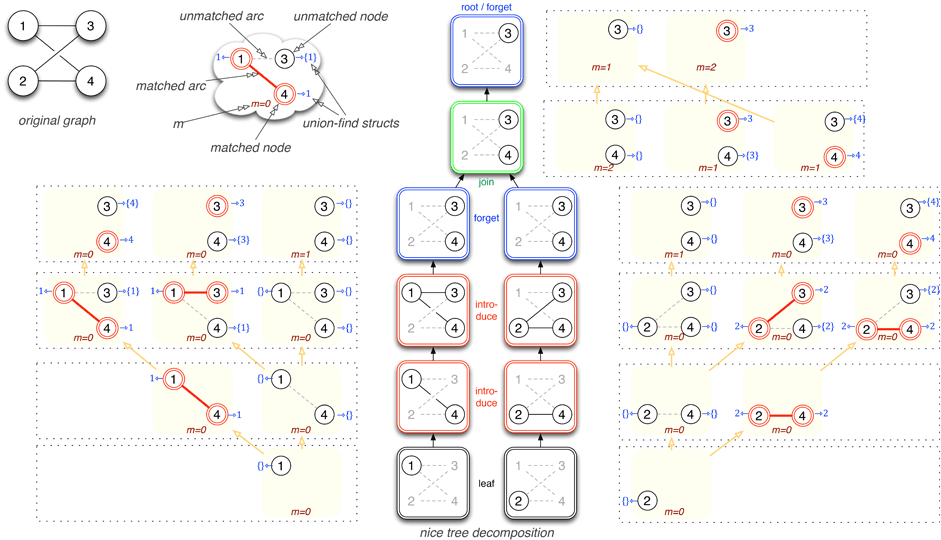## Parameterized Complexity of Discrete Morse TheoryBenjamin A. Burton, Thomas Lewiner, João Paixão, Jonathan Spreer

SoCG 2013 (ACM Transactions on Mathematical Software (TOMS) 42(1)): pp. no. 6 (february 2016)

## Abstract:

Optimal Morse matchings reveal essential structures of cell complexes that lead to powerful tools to study discrete geometrical objects, in particular, discrete 3-manifolds. However, such matchings are known to be NP-hard to compute on 3-manifolds through a reduction to the erasability problem. Here, we refine the study of the complexity of problems related to discrete Morse theory in terms of parameterized complexity. On the one hand, we prove that the erasability problem is W[P]-complete on the natural parameter. On the other hand, we propose an algorithm for computing optimal Morse matchings on triangulations of 3-manifolds, which is fixed-parameter tractable in the treewidth of the bipartite graph representing the adjacency of the 1- and 2-simplices. This algorithm also shows fixed-parameter tractability for problems such as erasability and maximum alternating cycle-free matching. We further show that these results are also true when the treewidth of the dual graph of the triangulated 3-manifold is bounded. Finally, we discuss the topological significance of the chosen parameters and investigate the respective treewidths of simplicial and generalized triangulations of 3-manifolds.

PDF paper (2.1 MB)## BibTeX:

@article{morse_complexity_toms,
author = {Benjamin A. Burton and Thomas Lewiner and João Paixão and Jonathan Spreer},
title = {Parameterized Complexity of Discrete Morse Theory},
year = {2016},
month = {february},
journal = {ACM Transactions on Mathematical Software (TOMS)},
volume = {42},
number = {1},
pages = {no. 6},
publisher = {ACM},
doi = {10.1145/2738034},
url = {\url{http://thomas.lewiner.org/pdfs/morse_complexity_toms.pdf}}
}

Last modifications on July 3rd, 2013